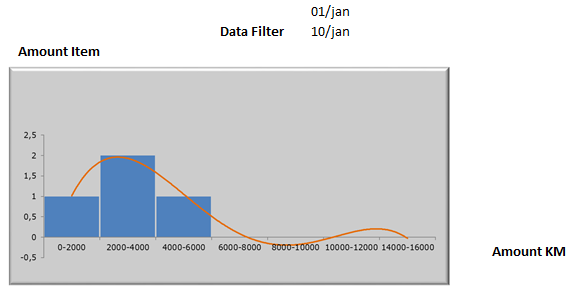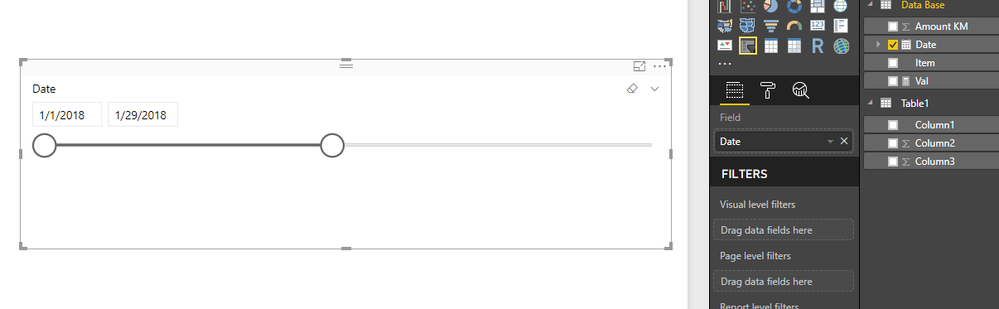cancel
Showing results for
Did you mean:Anonymous
Not applicable

## Variable Range based on date filter

Hello!

I'm new user of PBI and I'm trying to create a graphic were shows me the range of KM per Item based on a Data Filter.

The range I'm trying to get is:

1. >= 1.000 KM

2. Between 1001 to 2000 KM

3. Above 2001 KM

For exemple, if I select the Data Filter from 01/jan to 05/feb the range of item 1 must be 2. Between 1001 to 2000 KM ( 1.000 + 600 = 1.600). But if I select the Data Filter from 01/jan to 18/feb the range must be 3. Above 2001 KM (1.000 + 600 + 3.000 = 4.600).

 Data Base Item Date Amount KM 1 01/jan 1.000 2 02/feb 500 3 03/feb 600 4 04/feb 800 1 05/feb 600 2 12/feb 750 1 18/feb 3.000 2 20/feb 1.200 3 24/feb 1.600 4 26/feb 1.000 4 01/mar 1.600

The graphic I'm trying to create is something like this:Thanks!

1 ACCEPTED SOLUTIONMicrosoft

Hi @Anonymous,

Based on my test, you can refer to below steps:

1.Create a new table to record your range of amount KM.2.Create a measure to calculate the changed amount KM.

Val = var t=SUMMARIZE('Data Base','Data Base'[Item],"t7",CALCULATE(if(SUM('Data Base'[Amount KM])<=MAX('Table1'[Column2]) && SUM('Data Base'[Amount KM])>MIN('Table1'[Column3]),1)))

return

COUNTX(t,[t7])

3.Create a Table visual and add the [Val] and [Column1] field.4.Create a Slicer visual and add the [Date] filed.Now you can see the result.https://www.dropbox.com/s/eln6cm74539n2kl/Variable-Range-based-on-date-filter.pbix?dl=0

Regards,

Daniel He

Community Support Team _ Daniel He
If this post helps, then please consider Accept it as the solution to help the other members find it more quickly.
2 REPLIES 2Microsoft

Hi @Anonymous,

Based on my test, you can refer to below steps:

1.Create a new table to record your range of amount KM.2.Create a measure to calculate the changed amount KM.

Val = var t=SUMMARIZE('Data Base','Data Base'[Item],"t7",CALCULATE(if(SUM('Data Base'[Amount KM])<=MAX('Table1'[Column2]) && SUM('Data Base'[Amount KM])>MIN('Table1'[Column3]),1)))

return

COUNTX(t,[t7])

3.Create a Table visual and add the [Val] and [Column1] field.4.Create a Slicer visual and add the [Date] filed.Now you can see the result.https://www.dropbox.com/s/eln6cm74539n2kl/Variable-Range-based-on-date-filter.pbix?dl=0

Regards,

Daniel He

Community Support Team _ Daniel He
If this post helps, then please consider Accept it as the solution to help the other members find it more quickly.Anonymous
Not applicable

That's excactly what I need! Thank you !